# Galois theory facts for kids

Kids Encyclopedia Facts

Galois theory (pronounced gal-wah) is a subject in mathematics that is centered around the connection between two mathematical structures, fields and groups. Fields are sets of numbers (sometimes abstractly called elements) that have a way of adding, subtracting, multiplying, and dividing. Groups are like fields, but with only one operation often called addition (subtraction is considered addition with negative numbers). Fields are often hard to study because there are so many possible combinations of numbers, that the internal structure is hard to decipher. Groups are usually much less complex and easier to understand. Galois theory gives a concrete connection between hard to study fields and easy to study groups.

## Disclaimer

The nature of Galois' work is extremely advanced. It is usually considered out of reach for general audiences, or anyone without a strong technical background in Abstract algebra. This article will go into some detail, mostly defining types of equations and groups, and give a brief overview of the main ideas behind Galois theory. This article may not truly belong on Simple English Wikipedia, though it may be referenced by those who have trouble understanding the main Wikipedia page, and just need a brief discussion on the subject. Beyond the first paragraph of this page, some mathematical experience may be required. All things considered, any high schooler should be able to follow the main ideas laid forth in this article.

## Historical Motivation

### Euclid and Geometry

In the year 300 BCE, the Greek mathematician Euclid (pronounded yook-lid) wrote a book on geometry titled Elements. The text contained thirteen books, all on how to solve problems in geometry. Euclid was able to find ways to bisect any angle; that is, he was able to find an angle that was half of any other angle. Euclid was able to do much more, too. He could construct with a straightedge and compass shapes like circles, triangles, squares, pentagons, and others. However, he was unable to find a way to make every possible polygon. For example, Euclid was unable to make a regular 9-gon, a shape with 9 equal sides, using only ruler and compass. Mathematicians, like Euclid, tried to solve other problems as well. If they could cut any angle in two halves, then was there a way to cut any angle into three equal angles? Similarly, was there a way to square the circle (find a square with area equal to π, or a square with side length equal to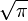$\sqrt{\pi}$ ), or double the cube(find a cube with twice the volume of another, by constructing a side of the cube with length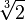$\sqrt{2}$ )? These questions remained unanswered for over 2000 years.

### The Sisters of the Quadratic Formula

A polynomial equation is an equation where you are only allowed to add and subtract multiples of powers of a variable, like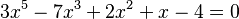$3x^5-7x^3+2x^2+x-4=0$. An nth power is a variable multiplied by itself n times. Or example polynomial has 5th, 3rd, 2nd, and 1st powers of x. The degree of a polynomial is the largest power found in the equation. In school, students learn about a type of polynomial equation called a quadratic equation. This is an equation where the highest power, or multiple, is two. An example of a quadratic equation is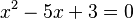$x^2-5x+3=0$, or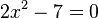$2x^2 - 7=0$. Notice that the only thing we care about is that there is a squared term. This makes the equation quadratic. If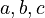$a,b,c$ are numbers and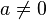$a \neq 0$, then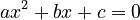$ax^2+bx+c=0$ is a valid quadratic equation. You may remember from school that if you know$a,b,c$ then you can solve this equation using the quadratic formula,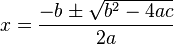$x = \frac{-b \pm \sqrt{b^2-4ac}}{2a}$which gives the solutions to our equation only by plugging in$a,b,c$. Mathematicians hoped to find a simple equation for the solutions (or, roots) for any polynomial equation, hopefully using only addition, subtraction, multiplication, division, and two other simple calculations: powers and roots. Mathematicians were able, after years of work, to find equations for polynomials with degree 3, and eventually degree 4. However, no one was ever able to find a formula for the solutions to a polynomial with degree 5 or higher. Why not? What was preventing them from being able to plug in the (in this case, since there are 5 powers)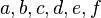$a,b,c,d,e,f$ in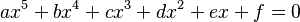$ax^5+bx^4+cx^3+dx^2+ex+f=0$ and get the answers? No one could find out why.

### Fields and Groups

The solution to these problems arrived out of the study of fields and groups. It turns out that each equation has a special field and group associated with it.

#### Fields

The field associated with each equation has complicated structure. However, you can tell a lot about the structure by how much you can rearrange elements by shuffling without changing the structure.

#### Groups and Sn

All of the ways of shuffling a field can be turned into a group. There are special types of groups, called the Symmetric Group on n elements labeled Sn, which are all the ways of shuffling n things. Symmetric groups are well behaved and easy to work with when you only shuffle 1, 2, 3, or 4 things. If you use 5 or more, the structure of the symmetric group becomes too chaotic.

## Galois's Solution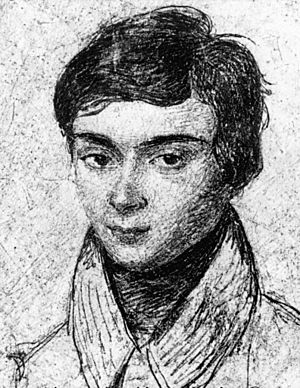Évariste Galois, who at the age of 18 formulated the theory named after him.

Up until the early 1800s, mathematicians were able to find some answers for specific cases of these unsolved problems. Unfortunately, no one had given a reason (or, proof) for any equation. It was French mathematician Évariste Galois (25 October 1811 – 31 May 1832) who was the first to find a solution, explaining why certain equations like 2nd, 3rd, and 4th degree polynomials did have nice solution formulas, but other equations, like 5th degree and above, cannot have a formula. The answer boiled down to reducing the problem of the equations's field to the equation's group; Galois proved that the two had a connection.

### Galois' Realization

Galois noticed that the structure of the groups associated with polynomials were really just the symmetric groups in disguise. If the degree of a polynomial was n, then the group of the polynomial was the symmetric group on n elements. Galois saw that the group of the equation shuffled the roots of the polynomial, and the structure of the shuffles could be analyzed instead.

### Solving Euclid's Problems

Euclid had wanted to find a way of cutting any angle in thirds. Galois showed that if a ruler and compass could be used to make a third of an angle, it would have an associated equation of degree 3. He showed that only powers of two could be made, by analyzing the equation's group and field. Galois showed that trisecting an arbitrary angle was impossible. Galois' theory could also be used to show that it was impossible to square a circle and double a cube.

### What Polynomials had Formulas?

Galois used his theory to also show that for polynomials of degree 5 and higher, their associated groups were Sn. He also showed that if an equation had a formula to solve it, then the group of the equation would have a nice structure and was not chaotic. Since the structure of the symmetric group is chaotic for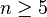$n\geq 5$, he showed that no general formula could exist for those polynomials.Galois theory Facts for Kids. Kiddle Encyclopedia.